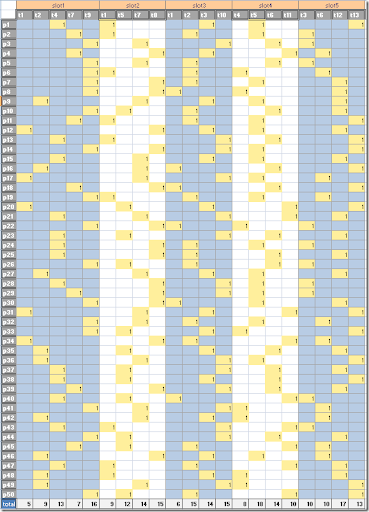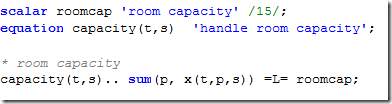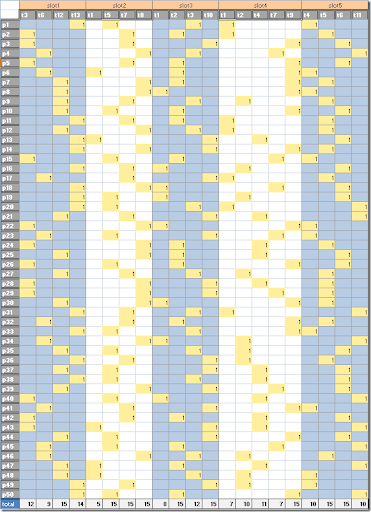## Friday, March 6, 2009

### A conference scheduling problem (2)

In this posting I described the solution for a conference scheduling problem. Now the question is posed: can we add a constraint on the room capacity: up to 15 people per room. The first thing I did was to inspect my solution. Indeed we are exceeding this limit here and there:In row total some of the occupancy counts are larger than 15. Note that this was a solution with objective=1491. We can add the room capacity constraint as follows:Using this additional constraint, the solution looks like:This reduces the optimal objective from 1491 to 1487. I.e. we pay for this with a small decrease w.r.t. the preferences. The solution time was 2 minutes with GAMS/Cplex.

I use here the fact that all rooms are identical. If there are five rooms with different capacity, we need to know how talks are assigned to individual rooms. That requires extra binary variables (either by adding a room index to variable x or by introducing a new variable that handles the room assignment).